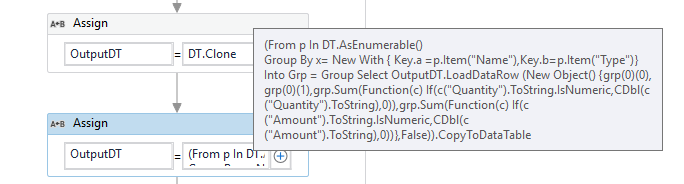# How to summarie tool the Data Table as follows

Hi Team .
i want to summarize the table and get the total amount and quantity as show below based on{ Name Type}

dose any one know the the method to do so ?

Thank you

The Operation that you are trying to Perform is a GroupBy and Sum. This can be done using an immediate Linq Expression as below :

``````(From p In DT.AsEnumerable()
Group By x= New With { Key.a =p.Item("Name"),Key.b=p.Item("Type")}
Into Grp = Group Select OutputDT.LoadDataRow (New Object() {grp(0)(0), grp(0)(1),grp.Sum(Function(c) If(c("Quantity").ToString.IsNumeric,CDbl(c("Quantity").ToString),0)),grp.Sum(Function(c) If(c("Amount").ToString.IsNumeric,CDbl(c("Amount").ToString),0))},False)).CopyToDataTable
``````

`OutputDT` is a Clone of the `DT` variable and have the same Datatype.

As it is shorter version of code, I have opted to use Linq. Please let us know if it is not the Expected answer you look for or if it doesn’t work as Expected.

You can use the Linq Expression in an Assign Activity as below :1 Like

@supermanPunch Thank you

1 Like

This topic was automatically closed 3 days after the last reply. New replies are no longer allowed.